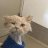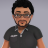# Scanner is not validating the condition for the entire set of bars

A

#### abhi

##### New member
I tried the below script in the Thinkorswim Scanner's thinkScript Editor to list the stocks whose 8EMA has not crossed over 21EMA over last 10 bars, but this is not giving me the expected result. Even if for one bar, the below condition is passing, the scanner is listing the stock. The scanner is not validating the condition for the entire set of 10 bars. Please correct me or provide me some pointers.

(MovAvgExponential("length" = 8)."AvgExp" crosses above MovAvgExponential("length" = 21)."AvgExp" ) is false within 10 bars

Last edited by a moderator:#### BenTen

Staff
VIP
Not crossing above means the 8 EMA is always below (less than) the 21 EMA. Try that instead.

A

#### abhi

##### New member
Thanks BenTen for your response. Even "is less than" is not giving the correct result. The problem seems that the if scanner finds the condition to be true for any of the bars, then it is listing the stock. The scanner is not validating the condition for every bar in the set. I am not sure if there is any keyword that instructs the scanner to validate the condition over all the bars (not just one single bar).

MovAvgExponential("length" = 8)."AvgExp" is less than MovAvgExponential("length" = 21)."AvgExp" within 10 bars#### BenTen

Staff
VIP
@abhi what if you adjust the timeframe? Say you want to apply that condition on the 5 min chart.

10 bars on the 5min chart would be roughly 1 bar on the 1H chart. Try scanning for the same condition but within 1 bar on the 1HR chart. Hope that makes sense.

A

#### abhi

##### New member
@BenTen, I am trying to learn the thinkScript, so for that purpose I need that information. Please respond if you have the answer for it, I would really appreciate that.#### MerryDay

##### Active member
VIP
Code:
``````def MA0 = MovAvgExponential("length" = 8)."AvgExp" is less than MovAvgExponential("length" = 21)."AvgExp" ;
def MA1 = MovAvgExponential("length" = 8)."AvgExp" is less than MovAvgExponential("length" = 21)."AvgExp" ;
def MA2 = MovAvgExponential("length" = 8)."AvgExp" is less than MovAvgExponential("length" = 21)."AvgExp" ;
def MA3 = MovAvgExponential("length" = 8)."AvgExp" is less than MovAvgExponential("length" = 21)."AvgExp" ;
def MA4 = MovAvgExponential("length" = 8)."AvgExp" is less than MovAvgExponential("length" = 21)."AvgExp" ;
def MA5 = MovAvgExponential("length" = 8)."AvgExp" is less than MovAvgExponential("length" = 21)."AvgExp" ;
def MA6 = MovAvgExponential("length" = 8)."AvgExp" is less than MovAvgExponential("length" = 21)."AvgExp" ;
def MA7 = MovAvgExponential("length" = 8)."AvgExp" is less than MovAvgExponential("length" = 21)."AvgExp" ;
def MA8 = MovAvgExponential("length" = 8)."AvgExp" is less than MovAvgExponential("length" = 21)."AvgExp" ;
def MA9 = MovAvgExponential("length" = 8)."AvgExp" is less than MovAvgExponential("length" = 21)."AvgExp" ;
def MA10 = MovAvgExponential("length" = 8)."AvgExp" is less than MovAvgExponential("length" = 21)."AvgExp" ;

plot scan = MA0 and MA1 and MA2 and MA3 and MA4 and
MA5 and MA6 and MA7 and MA8 and MA9 and MA10 ;``````

Copy and Paste this code as a study and in the scanner put scan equals true

•abhi and BenTen
A

#### abhi

##### New member
Thanks very much @MerryDay. I will try this code and will let you know incase I still have queries. I appreciate your support, efforts and time.

•MerryDayOn Balance Volume Scanner Questions 12Unusual Option Activity Scanner for ThinkorSwim Indicators 19